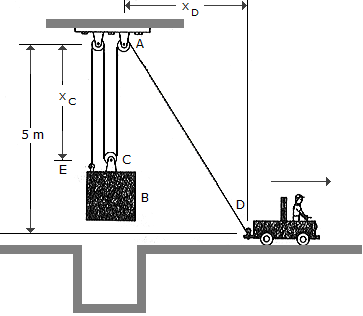# Engineering Mechanics - Kinematics of Particle (KOP) - Discussion

### Discussion :: Kinematics of Particle (KOP) - General Questions (Q.No.28)

28.The block B is suspended from a cable that is attached to the block at E, wraps around three pulleys, and is tied to the back of a truck. If the truck starts from rest when xD is zero, and moves forward with a constant acceleration of aD = 2 m/s2, determine the speed of the block at the instant xD = 3 m.

 [A]. vB = 0.1715 m/s [B]. vB = 1.155 m/s [C]. vB = 0.594 m/s [D]. vB = 0.515 m/s

Explanation:

No answer description available for this question.

 Jim said: (Feb 18, 2017) How? Explain.

 Bhokaal said: (Apr 30, 2017) Find the velocity of a truck at Xd=3 which is root12 then find the velocity of string AD which is 3root (12/34). So, the velocity of the block will be a velocity of AD divided by 3, hence answer is 0.594.

 Sonakshi said: (Sep 4, 2018) At instant 3 xb+root of xd ^2 +16=L. 3vb+xd/ √16+xd^2 dxd/dt=0. So,vb=0.6m/s.